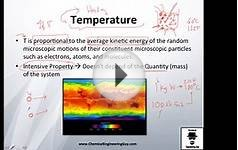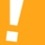# Simple definition of second law of thermodynamics

5.1 Concept and Statements of the Second Law (Why do we need a second law?)

• No process is possible whose sole result is the transfer of heat from a cooler to a hotter body. [Clausius statement of the second law]
Figure 5.2:For , this is not possible (Clausius)
• There exists for every system in equilibrium a property called entropy, , which is a thermodynamic property of a system. For a reversible process, changes in this property are given by

The entropy change of any system and its surroundings, considered together, is positive and approaches zero for any process which approaches reversibility.

For an isolated system, i.e., a system that has no interaction with the surroundings, changes in the system have no effect on the surroundings. In this case, we need to consider the system only, and the first and second laws become:

For an isolated system the total energy is constant. The entropy can only increase or, in the limit of a reversible process, remain constant.

The limit, or , represents the best that can be done. In thermodynamics, propulsion, and power generation systems we often compare performance to this limit to measure how close to ideal a given process is.

All of these statements are equivalent, but gives a direct, quantitative measure of the departure from reversibility.

Entropy is not a familiar concept and it may be helpful to provide some additional rationale for its appearance. If we look at the first law,

the term on the left is a function of state, while the two terms on the right are not. For a simple compressible substance, however, we can write the work done in a reversible process as , so that

Two out of the three terms in this equation are expressed in terms of state variables. It seems plausible that we ought to be able to express the third term using state variables as well, but what are the appropriate variables? If so, the term should perhaps be viewed as analogous to where the parentheses denote an intensive state variable and the square brackets denote an extensive state variable. The second law tells us that the intensive variable is the temperature, , and the extensive state variable is the entropy. The first law for a simple compressible substance in terms of state variables is thus

 (5..1)

Because Eq. includes the second law, it is referred to as the combined first and second law. Because it is written in terms of state variables, it is true for all processes, not just reversible ones.

You might also likeDefinition of Temperature // Thermodynamics - Class 3Physics - Thermodynamics: (1 of 5) Entropy - Basic Definition

### FAQ##### Biology. /. Second Law Thermodynamics/Entropy.

Good question Chastity.  The Law of entropy states that with any change of energy from one form to another there is a loss of useful energy.  Life does not violate this tenet.  In a living system the 2nd law is continually in force during the metabolic process.  When the process ceases (death) the cell return to order as each component breaks down to the individual atoms. (dust to dust.
For a better explanation go here: